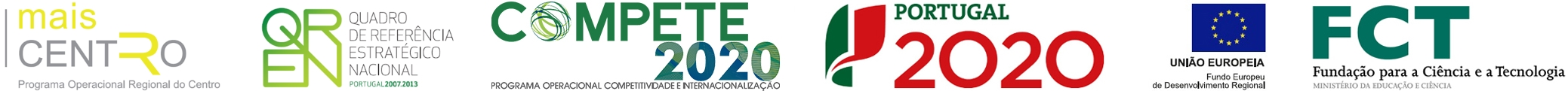Thermo-physicochemical investigation of molecular interactions in binary combination (dimethyl carbonate plus methyl benzoate)
 authors Jyothirmai, G; Nayeem, SM; Khan, I; Anjaneyulu, C nationality International journal JOURNAL OF THERMAL ANALYSIS AND CALORIMETRY author keywords Density; Sound speed; Excess parameters; Prigogine-Flory-Patterson theory; Theory of topology; SRK/PR EoS; Activity coefficients; Nonlinear equations keywords EQUATION-OF-STATE; LIQUID-MIXTURES; THERMODYNAMIC PROPERTIES; PHYSICAL-PROPERTIES; EXCESS PROPERTIES; 308.15 K; TOPOLOGICAL ASPECTS; REFRACTIVE-INDEXES; DIETHYLENE GLYCOL; ETHYL BENZOATE abstract To probe the nature of interaction and its deeds with temperature in the binary combination (dimethyl carbonate + methyl benzoate), the density (rho)/speed of sound (u) be established for the total mole fraction array at T = (308.15, 313.15 and 318.15) K and at atmospheric pressure. Sign with magnitude of evaluated excess molar volume (V-m(E)) was inspected and comprehensive analysis elucidated not only pervasiveness of strong molecular interactions between molecules but also its transform with temperature. Additional, strong interactions are properly hold up by partial and excess partial molar volume ((V) over bar (m), (V) over bar (E)(m), (V) over bar (E,infinity)(m)). Additionally, relative association (R-A), Lennard-Jones repulsive power (n), excess isentropic compressibility (K-s(E)), partial and their excess partial molar isentropic compressibility ((K) over bar (m), (K) over bar (E)(m)), excess values of isobaric thermal expansion coefficient (alpha(E)(P)), isothermal compressibility (k(T)(E)), intermolecular free length (L-f(E)), acoustic impedance (Z(E)), ultrasonic speed (u(E)) were evaluated and confirmed the deductions of V-m(E). By Redlich-Kister equation, standard deviations are computed through coefficients for excess parameters. At T = 308.15 K, V-m(E) is correlated by theories of Prigogine-Flory-Patterson (PFP)/topology/Soave-Redlich-Kwong (SRK)/Peng-Robinson (PR) cubic equation of states; prophesied first-order derivatives of thermodynamic potentials; using semi-empirical equations, excess chemical potential/activity coefficients/theoretical speeds are estimated and correlated. Further, microscopic molecular properties are assessed at all temperatures by Sehgal's equations on nonlinear relations. publisher SPRINGER issn 1388-6150 year published 2018 volume 132 issue 1 beginning page 693 ending page 707 digital object identifier (doi) 10.1007/s10973-017-6926-8 web of science category Thermodynamics; Chemistry, Analytical; Chemistry, Physical subject category Thermodynamics; Chemistry unique article identifier WOS:000426795200066
ciceco authors
impact metrics
 journal analysis (jcr 2019): journal impact factor 2.731 5 year journal impact factor 2.325 category normalized journal impact factor percentile 58.156
 dimensions (citation analysis): altmetrics (social interaction):

Apoio